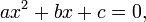# Physics Motions Questions: Additional Information- Sept. 9, 2008

After looking at the questions in the text, I realized that there are a few equations you might need that you may or may not have encountered yet. The main equation that is needed seems to be the quadratic equation.$ax^2+bx+c=0,\,\!$$x = \frac{-b \pm \sqrt {b^2-4ac}}{2a},$

Whenever you have some form of an equation that resembles the top equation, you can solve for your unknown using the second formula. a, b, and c represent some numerical coefficient. You will be left with two possible values of x; verify which x makes sense (example: negative time makes no sense so is an invalid number). In the context of our questions, we can use the formulae about to solve for t.